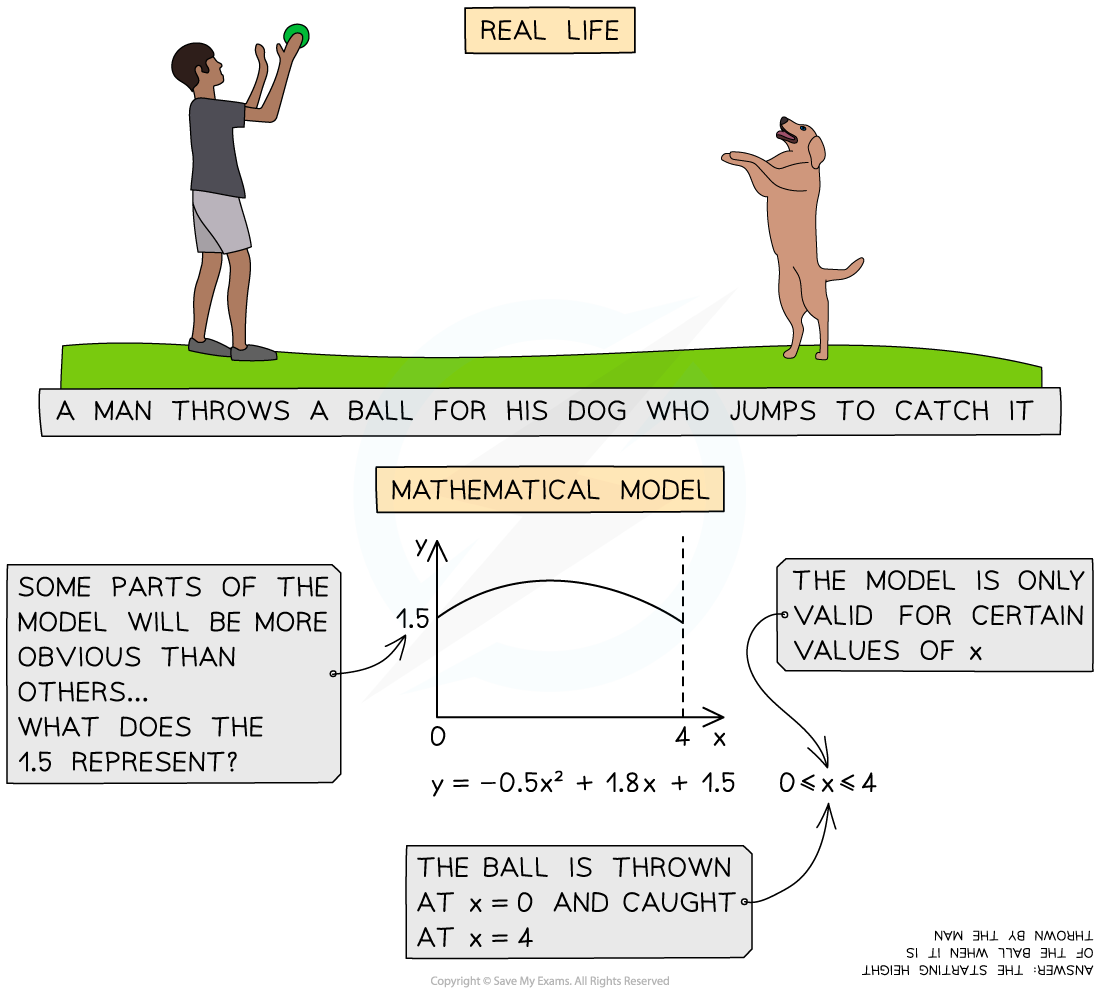# CIE A Level Maths: Mechanics复习笔记1.1.5 Modelling Assumptions

### Modelling Assumptions

#### What is modelling in Mechanics?

• Mechanics uses modelling to solve problems in real life situations
• We use assumptions to simplify real life problems in order to turn them into equations or graphs that can be solved
• We will sometimes need to criticise or refine our assumptions to improve the model#### Modelling Assumptions in Mechanics:

• There are many different modelling assumptions that can be made when dealing with real life situations
• Different assumptions will be needed for different models
• The assumptions you make will affect the calculations in each problem differently

#### What types of modelling assumptions are there?

• Gravity is constant and vertical
• Air resistance is usually modelled as negligible and can be ignored
• smooth surface has no friction
• rough surface has a frictional force between the surface and any object that makes contact with it
• particle has negligible dimensions, therefore forces will all act on a particle at the same point
• rod or a beam should be treated as a long, rigid particle
• uniform object’s mass is distributed evenly
• light object has zero mass
• An inextensible object cannot be stretched

#### Worked Examplea) A and B are both particles.

b) The string is light.

c) The string is inextensible.

d) The pulley is smooth.

e) The surface A is moving along is smooth.#### Exam Tip

• Make sure you fully understand the definitions of all the words in this section so that you can be clear about what your exam question is asking of you.